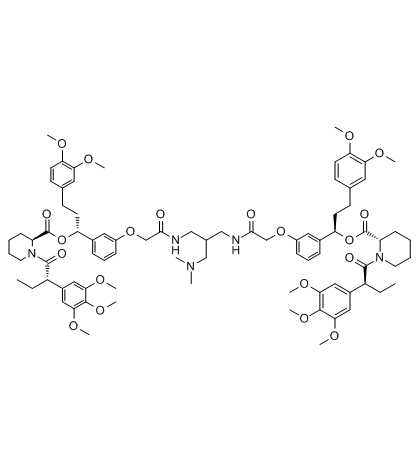AP20187

常用名 英文名 CAS号AP20187结构式 AP20187 AP20187 195514-80-8 1482.75000 N/A N/A C82H107N5O20 N/A N/A N/A

AP20187用途

AP20187 是一种细胞渗透性分子，使 FK506 结合蛋白 (FKBP) 二聚化，启动生物信号传导级联反应和基因表达，或破坏蛋白-蛋白之间相互作用。

AP20187名称

中文名 AP20187 ap20187

AP20187生物活性

FKBP homodimerizer

DMSO：≥57mg / mL（38.44 mM）

* “≥”表示可溶，但饱和度未知。

 1 mM 0.6744 mL 3.3721 mL 6.7442 mL 5 mM 0.1349 mL 0.6744 mL 1.3488 mL 10 mM 0.0674 mL 0.3372 mL 0.6744 mL

 -20℃下 4℃下 粉末 3年 2年 在溶剂中 6个月 1个月

SMILES COC1=C(OC)C=C(CC[C@H](C2=CC=CC(OCC(NCC(CN(C)C)CNC(COC3=CC=CC([C@H](OC([C@H]4N(C([C@@H](CC)C5=CC(OC)=C(OC)C(OC)=C5)=O)CCCC4)=O)CCC6=CC(OC)=C(OC)C=C6)=C3)=O)=O)=C2)OC([C@@H]7CCCCN7C([C@H](C8=CC(OC)=C(OC)C(OC)=C8)CC)=O)=O)C=C1

*以上文档由Medchemexpress提供，仅用于科学研究参考。

AP20187物理化学性质

分子式 C82H107N5O20 1482.75000 1481.75000 265.42000 11.88220

AP20187生产厂家